# Generalizing the yield function

Home » Knowledge Base » General » Materials » Generalizing the yield function

The linear yield function given σ y = σ 0 y + h ε^-p is too simple for many applications. A more general, nonlinear form, σ y( ε^p; ε^p; …) is easily incorporated within the radial return framework. The only two places where the yield function appears in the previous derivation are in determining if plastic ow occurs, Equation 4, and the discrete consistency condition for determining the increment in plastic strain, Equation 7.

Equation 4 generalizes to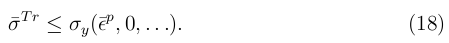where the plastic strain rate is assumed to be zero to be consistent with the assumption that the update is elastic.

In a similar manner, Equation 4 generalizes to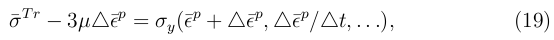giving a nonlinear equation that may be solved iteratively. From continuum mechanics, we know that the increment in plastic strain lies somewhere between zero and the entire strain increment, Δ ε . The increment in equivalent plastic strain, therefore, must lie somewhere between 0 and sqrt((2/3) Δ ε : Δ ε), and the equation may be solved with an iterative method that is guaranteed to converge (eventually).

The yield stress of many materials is a function of the temperature, T, with the yield strength decreasing as the temperature increases. Heat transfer, and the work associated with the stress, alter the temperature. Most finite element analyses that couple heat transfer with the mechanical solution do so loosely, that is, the geometry is held fixed during the heat conduction and there is no heat conduction during the mechanical equilibrium solution. Discussing the coupling algorithms is beyond the scope of the present discussion, but extending Equation 19 to include the effects of temperature through the plastic work is not difficult.

From the energy equation, the temperature evolves (to second order accuracy) according to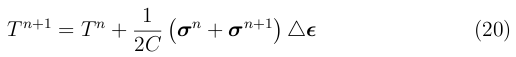where C is the heat capacity. Assuming that the step is plastic, the temperature evolution may be approximated to first order accuracy as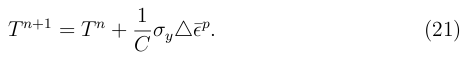Equation 19 becomes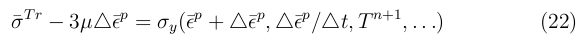where T^n+1 is updated using the values from the previous iteration.

When thermal softening occurs, the increment in plastic strain can exceed the total strain increment. Iterative methods that require a bounding interval for the solution may fail in this situation if the upper bound on the equivalent plastic strain is based on the total strain increment. Since the final yield stress is greater than, or equal to, zero, the increment on equivalent plastic strain is bounded by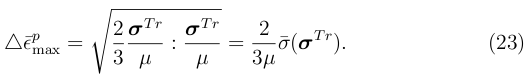djb 2005